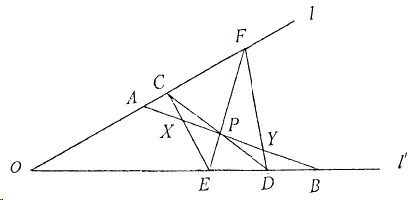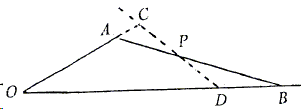# Butterfly with Menelaus

### Sidney Kung September 7, 2012

We give a proof of the following by using Menelaus' theorem:

Through a point $P$ of the line segment $AB$ whose end points $A$ and $B$ lie on two intersecting lines $l$ and $l'$, respectively, draw $CD$ and $EF$ $(C, F\in l$, and $E,D\in l')$. Let $CE\cap AB=X$, $FD\cap AB=Y.\space$Then $PA = PB$ implies $PX = PY.$### Proof

For a repeated application of Menelaus' here, we shall proceed by focusing on parts of the diagram:

1. $\triangle OFE$ cut by transversal $CPD$$\frac{OC}{CF}\times \frac{FP}{PE}\times \frac{ED}{DO} = 1$.

2. $\triangle OAB$ cut by transversal $CPD$$\frac{OD}{DB}\times \frac{BP}{PA}\times \frac{AC}{CO} = 1$.

3. $\triangle PBE$ cut by transversal $FYD$$\frac{PY}{YB}\times \frac{BD}{DE}\times \frac{EF}{FP} = 1$.

4. $\triangle FAP$ cut by transversal $CXE$$\frac{PE}{EF}\times \frac{FC}{CA}\times \frac{AX}{XP} = 1$.

Multiplying the four identities and simplifying gives $\frac{PY}{YB}\times \frac{AX}{XP}\times \frac{BP}{PA} = 1$. Since $PB=PA$, the latter expression further simplifies to $\frac{AX}{XP}=\frac{YB}{PY}$. Add now $1$ to both sides: $\frac{AX+XP}{XP}=\frac{PY+YB}{PY}$, or $\frac{PA}{XP}=\frac{PB}{PY},\space$implying $PX=PY$.The above proof moved Hubert Shutrick to make the following observation:

The conics of the pencil through $C$, $D$, $E$, $F$ intersect the line $AB$ in pairs of points in involution. Consider the degenerate members $CD.EF$, $CE.DF$, and $l.l'$. $P$ is a double point because of the first and so, if one of the pairs $A,B$ and $X,Y$ is symmetrical about $P$, then so is the other.### Butterfly Theorem and VariantsCopyright © 1996-2018 Alexander Bogomolny

 65271853